All submissions of the EM system will be redirected to Online Manuscript Submission System. Authors are requested to submit articles directly to Online Manuscript Submission System of respective journal.

# Opinion Review

, Volume: 11( 1) DOI: 10.37532/2320-6756.2023.11(1).320

## A Theory On Black Hole Dynamics

*Correspondence:
Independent Researcher, Journal of Physics and Astronomy, India
E-mail:
[email protected]

Received date: 19-January-2023, Manuscript No. tspa-23-87784; Editor assigned: 21-January-2023, Pre-QC No. tspa-23-87784 (PQ); Reviewed: 27-January-2023, QC No. tspa-23-87784 (Q); Revised: 28-January-2023, Manuscript No. tspa-23-87784 (R); Published: 31-January-2023, DOI. 10.37532/2320-6756.2023.11 (1).320

Citation: Sarkar NS. A Theory on Black Hole Dynamics.2023;11(1):320.

### Abstract

I basically showed in this paper that the luminosity of a black hole is inversely proportional to the square of its mass. By applying some simple math we can relate mathematical properties to each other very easily. My paper says that the lower the mass of the black hole, the higher its luminosity. This mass-luminosity relation is completely different from the Eddington mass-luminosity relation. This means that if a black hole has less mass, its luminosity will be higher. But if the mass of the black hole is more, its luminosity will be less. I explained it this way, in the case of a low mass black hole, most of its mass is converted into energy. For which its mass is less. So its luminosity is high, and it’s also the reason of low surface area. But in case of black hole with more mass, its surface area will be more. So with more surface area, it becomes more likely that more anti-matter will enter there through Quantum Fluctuations. And it will reduce its mass energy density. If the mass density decreases, the mass will decrease. For which he will not get sufficient mass, so that he can eject an intense radiation. Let’s describe it again. The density of a small black hole is much higher, so its gravitational pull is much higher. It will then begin to consume large amounts of matter. Try to consume as much matter as possible. As a result, there will be a possibility to increase its size. And converting most of the ingested matter into energy. As a result, its luminosity will be higher. But in the case of a large black hole, the density is not high. It cannot be more compact than any small black hole. So its gravitational pull will be considerably less. It cannot consume more matter through the accretion disc. Since the black hole is bigger in terms of size, its surface area will be more. Having more surface area means that the possibility of anti-matter entering it or interacting with the surface will also increase a lot. And it's all quantum mechanically happened. Anti-matter will reduce the mass density of the black hole. As a result, the energy of the black hole will decrease. Its size will gradually disappear. Its entropy will decrease. Once the black hole will evaporate through hawking radiation.

### Keywords

Black Holes; Mass; Luminosity; Temperature; Energy; Supernova

### Introduction

Black holes are one of nature's strangest objects. At the end of its life, a star explodes in a supernova, releasing all its material into space. But the stars don't end there. The core of the star still remains. After a supernova explosion, if a white dwarf star has a mass greater than 1.4 solar masses, the neutron degeneracy pressure cannot prevent the star's gravitational contraction. The star continues to contract. At some point it reaches the boundary line which is called event horizon. That is, the star turns into a black hole. It is impossible to predict what is happening inside the event horizon. This event horizon separates the black hole from our world. Any object that enters the event horizon is cut off from the outside world. Once an object enters the event horizon, it can never re-exit through it. Because to do that, matter has to travel faster than the speed of light. However, any object beyond the event horizon of a black hole can easily travel away from light or even at speeds less than light. But somehow, once light enters the Horizon, it cannot escape the black hole's gravity. At the center of the black hole is a singularity, where gravity becomes infinite, since then r=0. Space and time have no meaning in singularity. A disc of ionized gas is observed around the black hole, called the "accretion disc". Along this disc, the black hole swallows other stellar objects. As the mass of the black hole increases, its volume also increases. As the mass of the black hole increases, the temperature decreases. According to my calculations, it appears that black holes that are more luminous have less mass. But those black holes which are less luminescent, have comparatively more mass. Moreover, black holes that have lower temperatures have significantly larger masses. But those whose temperature is high, their mass is low. We can define the temperature of an object as the amount of heat contained in that object. Now heat is energy. And its square is proportional to the luminosity of the black hole. Besides, it is known from Benkenstein formula that entropy and surface area of black hole are proportional to each other. The greater the surface area of the black hole, the greater its entropy. There is only so much chaos in it. In my mathematical theory, I first imagined a light wave, where the wave length is equal to the radius of the black hole. This radius is equal to the Schwarzschild radius. This particular radius represents the minimum size a black hole can grow. Then I put this radius, E=hc/λ in place of λ. So that I can get the energy expression. And that's pretty elegant. I applied the Stefan Boltzmann formula (but only for Schwarzschild black holes). Where the squared value of R(s) is substituted for the square of the radius. And the value of "T=hc³/2MG" is put in place of temperature. Finally I noticed a mathematical relationship between Luminosity and Mass. From the Planck relation or Planck-Einstein relation, we more or less learned that; the energy of a photon can be described by this formula.or,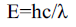As, ν = c/λ

Here, ν=Frequency of the light (photon).

h=planck constant.

c= Speed of light

Let’s considered, the radius of the Black hole equals to the wavelength of the photon that falls into the black hole, and one photon has one unit of entropy.

So, R(s)=λ,

R(s) is the minimum radius of the black hole of mass M.

Now put this special case into the equation E=hc/λ, so we will get;And what is R(s)?

It’s the Schwarzschild radius of black hole, where R(s) equals to;Put this R(s) value in the equation 1, we might be get;or,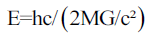or,Now, Energy is also the measurement of Temperature times Boltzmann constant.k=Boltzmann Constant

or,or,Black holes are also like stars. They have Temperature, Mass, Spin, Gravity, Radiation, and all the crazy stuffs that a star needed. Black holes eject light or electromagnetic radiation in the form of Quasars. A quasar is an extremely luminous active galactic nucleus, powered by a black hole, with mass ranging from millions to tens of billions of solar masses, surrounded by a gaseous accretion disc. Probably the disc made by a vast amount of photon Gases. Gas in the disc falling towards the black hole heats up because of friction and releases energy in the form of electromagnetic radiation. So, they have certain Luminosity. Now we apply Stefan-Boltzmann law for a Schwarz’s child black hole luminosity. If this law is applicable for stars, then it also applicable for black holes. Moreover black holes are made when a star run out of its nuclear fuel, and collapses due to the effect of gravity. The law is,Here,

L(B×H)=Luminosity of the black hole.

σ=Stefan-Boltzmann Constant.

T=Temperature Of the black hole.

Now, R(s)=2MG/c²

And, T=hc³/2MkG

Putting this two value in the equation 4, we must get: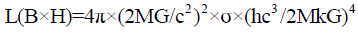or,or,or,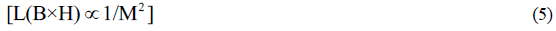So, the luminosity of a black hole inversely proportional to the square of its mass. That mean if a black hole is massive, then it is less luminous. But if the black hole has less mass then it is much luminous. Moreover, Temperature is inversely proportional to the Mass (M). So;If we relate this expression with "equation 5", then we will get,The above equation shows, if the black hole has less temperature, then it also has less luminosity, but if the black hole has much temperature then it has a vast amount of luminosity. By the way, what is the definition of temperature? Temperature is the amount of heat that stored in an object. Heat is energy. So the more luminous a black is, the more energy it has. It is consistent with Temperature. Now take a further step. I want to calculate the density of the Black hole. First of all black holes are spherical in shape and size. So the density of a sphere is ρ=M/V.

For a Black hole it will be;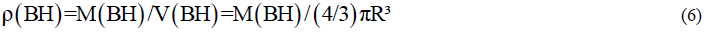As we knew that M(BH)=1/T, and R=2M(BH)G/c², T is the temperature of the Black hole. Here I neglect the constant parts that is not our main point. If I put the value of R in the equation I shall get.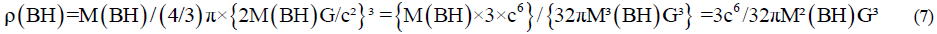Let’s neglect the constant part of this above equation. By the way, BH Stands for Black Hole.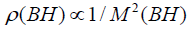As we knew that M(BH)=1/T, so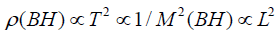If the temperature of a Black hole is high then it is very dense, that mean mass is not too much,(matters are packed into very small regions, due to gravitational construction, and that’s why it has large density), but very luminous. So, Black hole has Luminosity, Black hole has Temperature, Black hole has entropy, And the expression are given below: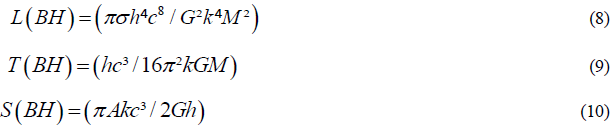From equation 9 we can also say:From equation 8, we rewrite this expression,But From equation 10:If I put this value for 1/M in equation 8, I shall get: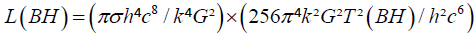For getting Entropy we can do this below assumption: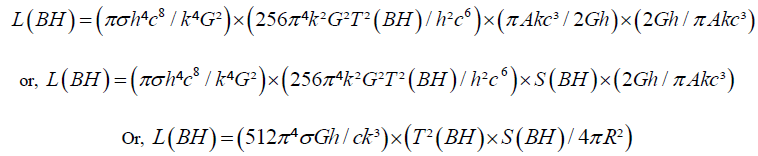As Area A=4πR², and R=2MG/c²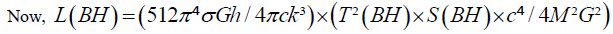or,or,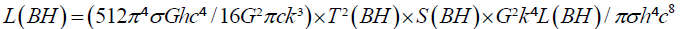or,or,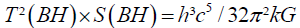or,Now I put the value of the Constants in the above equation, so the final Expression will be:or,or,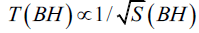So the lower the entropy of the black hole, the higher its temperature. This is the relationship between the temperature and entropy of a black hole. Moreover, this above equation refers to consistency of luminosity equation. If the luminosity equation was wrong, then the Entropy-Temperature relation could never be deduced from it. Since the luminosity relation is used to determine it.

Welcome Message

Select your language of interest to view the total content in your interested language

###### Citations : 260

Journal of Physics & Astronomy received 260 citations as per Google Scholar report

#### Indexed In

• Open J Gate
• China National Knowledge Infrastructure (CNKI)
• Cosmos IF
• Directory of Research Journal Indexing (DRJI)
• MIAR
• Secret Search Engine Labs
• Euro Pub
• clarivate-web-of-science-logo-vector

### 9th International Conference on Physics

Edinburgh, Scotland

### 6th International Conference on Astronomy, Astrophysics and Space Science

Budapest, Hungary

Berlin, Germany

### 9th International Conference on Physics

Edinburgh, Scotland

### 6th International Conference on Astronomy, Astrophysics and Space Science

Budapest, Hungary

### World Congress on Quantum Physics

Berlin, Germany

#### For Librarians#### Open Access Journals